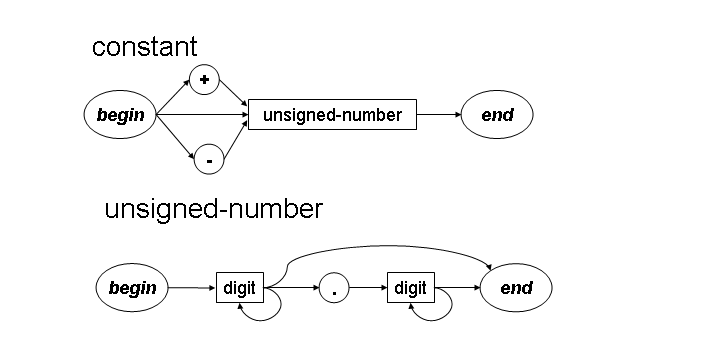University of Virginia, Department of Computer Science CS200: Computer Science, Spring 2002

 Exam 1 Out: 25 February 2002 Due: 27 February 2002, 11:00AM

Work alone. You may not discuss these problems or anything else related to this course with anyone except for the course staff between receiving this exam and class Wednesday. You may send email to cs200-staff@cs.virginia.edu to ask for clarifications on what the questions mean.

Open book. You may use any books you want, lecture notes and slides, your notes, and problem sets. If you use anything other than the course books and notes, cite what you used.

Closed web. You may not search the web for material relevant to this problem set.

No DrScheme. You may not run DrScheme or use any other Scheme interpreter between now and when you turn in this exam.

Answer well. Answer all 7 question. Write and number your answers clearly. Staple the sheets you turn in and put your name on them. Full credit depends on the clarity and elegance of your answer, not just correctness. Your answers should be as short and simple as possible, but not simpler.

1. Describe the language defined by this recursive transition network using Backus-Naur Form:A digit is 0, 1, 2, ..., 9. The symbols inside circles (., +, and -) are terminals. Your Backus-Naur Form grammar should not use the * shortcut notation. For full credit, your grammar should be the shortest possible grammar that describes the same language.

2. Ben Bitdiddle suggests implementing length, a procedure that takes a list as a parameter and evaluates to the number of elements in the list, by first replacing every element in the list with 1, and then summing the list. You may assume you have a procedure sumlist that takes a list of numbers and evaluates to the sum of all the numbers in the list.

For example, we can determine the length of the list (list 1 2 3 "alpha" "beta") by constructing the list (list 1 1 1 1 1) and evaluating (sumlist (list 1 1 1 1 1)) to get 5.

Ben started to define his length procedure below, but couldn't figure out what to put in the space where the box is. Finish the definition of length by providing a Scheme expression for the missing parameter to map:

```   (define (bens-length lst)
(sumlist
(map _________________________________________ lst)))
```

3. Louis Reasoner suggests that a general procedure to make a constant function would be useful for defining functions like bens-length. That is, he would like a procedure that takes one parameter, and evaluates to a procedure that produces that value when applied to anything. For example,

```> ((make-constant-function "apple") "orange")
"apple"
> (((make-constant-function (make-constant-function 12)) "orange") 35)
12
```
Define make-constant-function.

4. The bens-length procedure (and the Scheme primitive length) counts only the elements in the top-level list. For example, (bens-length (list (list 1 2 3) 4)) ==> 2 since the inner list (list 1 2 3) only counts as one element. Alyssa suggests defining a length-deep procedure that evaluates to the total number of elements in a list and all its sub-lists. For example, (length-deep (list (list 1 2 3) 4)) ==> 4 and (length-deep (list (list (list 1 2) (list 3 4) (list 5 (list 6 7)))) ==> 7. Alyssa claims she can define length-deep using only these Scheme primitives: null?, list?, +, 0, 1, car and cdr. Define length-deep, a procedure with one list parameter that evaluates to the total number of elements in the list and all its sub-lists.

5. Define a procedure reverse that takes a list parameter and evaluates to a list containing the elements of the original list in reverse order. You may find it useful to define extra procedures also. You may not use the Scheme primitive reverse procedure. For this question, do not use insertlg. Example:

```> (reverse (intsto 5))
(5 4 3 2 1)
```
6. Define reverse (with the same meaning as the previous question) using insertlg.

7. Orders of Growth

1. How much work is your reverse procedure from Question 5.
2. How much work is your reverse procedure from Question 6.
Express your answers using Θ and n for the length of the list. Assume cons, car, cdr, and null? take constant time (that is, the time they take does not depend on the length of the list).